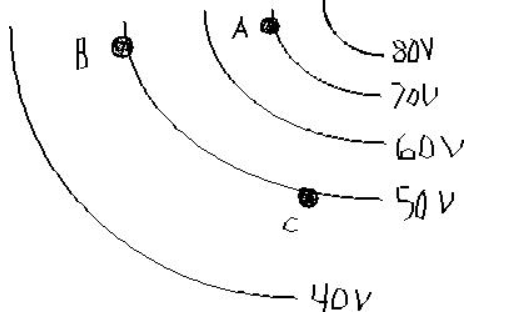# Problem: Three points (A, B, and C) are located on equipotential lines as shown.1. A proton is released from Point A. Indicate the direction of the electric force vector acting on the proton.The electric force vector at Point A points in which direction?a) upb) downc) leftd) righte) is zero2. The electric force vector at PointB points in which direction?a) upb) downc) leftd) righte) is zero3. An electron is released from Point B and a second electron is released from Point C. What can you say about the electric forces experienced by these electrons the instant they are released?a. The electron released at Point B experiences a greater force.b. The electron released at Point C experiences a greater force.c. Electrons released from Points B and C would experience equal forces.d. Therelationship between the two forces cannot be determined.

###### FREE Expert Solution

We need to master some concepts before tackling this problem.

One of them is the expression for work, which is:

$\overline{){\mathbf{W}}{\mathbf{=}}{\mathbf{-}}{\mathbf{q}}{\mathbf{∆}}{\mathbf{V}}}$, where is charge and ΔV is the potential difference.

The potential difference ΔV for equipotential surfaces is zero.

ΔV = 0.

This means that W = 0.

The electric force is expressed as:

$\overline{){\mathbf{F}}{\mathbf{=}}{\mathbf{q}}{\mathbf{E}}}$, where E is the electric field.

The electric field and potential are related by:

$\overline{){\mathbf{E}}{\mathbf{=}}{\mathbf{-}}\frac{\mathbf{d}\mathbf{V}}{\mathbf{d}\mathbf{x}}}$, where V is the electric potential.

93% (230 ratings)###### Problem Details

Three points (A, B, and C) are located on equipotential lines as shown.1. A proton is released from Point A. Indicate the direction of the electric force vector acting on the proton.
The electric force vector at Point A points in which direction?
a) up
b) down
c) left
d) right
e) is zero

2. The electric force vector at PointB points in which direction?
a) up
b) down
c) left
d) right
e) is zero

3. An electron is released from Point B and a second electron is released from Point C. What can you say about the electric forces experienced by these electrons the instant they are released?
a. The electron released at Point B experiences a greater force.
b. The electron released at Point C experiences a greater force.
c. Electrons released from Points B and C would experience equal forces.
d. Therelationship between the two forces cannot be determined.